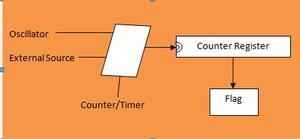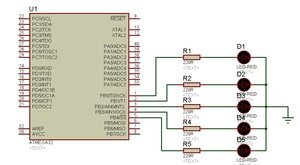# how to use timers of avr microcontroller

A Timer is actually a counter that is increased every time when an instruction is executed. There are one to six timers in AVR Microcontrollers depending upon their family architecture.The AVR timers are of 8 bits and 16 bits. The 8-bit timers are termed as TCNTn while the 16 bit timers are termed as two 8 bit registers (TCNTnH, TCNTnL).Generally AVR like ATmega32 consists of three timers Timer0, Timer1 and Timer2 respectively. The timer0 and timer2 are 8 bit registers whereas the timer1 is a 16 bit register.   Every timer has its own TCCR (Timer/Counter Control Register) register that is responsible to choose different operational modes.

### Timers of AVR microcontroller

Timers can be used to generate time delays or as a counter to count events.If we want to use timer as counter then we can join the external source to the clock pin of counter register. The content of the counter is incremented when an event is occurred. This increment shows that how many times an event has occurred. Now if we want to generate time delays then we can connect the oscillator to the clock pin of counter register. This results an increment in the content of the counter when an oscillator ticks. This shows that how many ticks have been occurred when cleared the counter. We can easily calculate the tick period as the speed of oscillator is known and we will recognize how much time has been over and done from the content of the register.

There is also a flag for each counter in the microcontrollers. When the counter overflows the flag is set. The other technique to generate time delays is to load the counter register and wait for the time when the overflow of the counter takes place and flag is set. For example, in a microcontroller with a frequency of 1MHZ using 8-bit counter register, if we need a time delay of 2 seconds we just have to load the counter register with \$FE and wait until the flag is set. In this case, after the first tick the content of the clock register increases to \$FF and after the second tick it overflows. In other words, the content of the register becomes \$00 and the flag is set. This expression is illustrated in the following diagram.### Basic registers of timers

There is a TCNTn called timer/Counter Register for each of the timers in AVR. For example, In Atmega32 we have TCNT0, TCNT1 and TCNT2.  The TCNTn is basically a counter. It counts up with each pulse. It contains zero when reset. We can read or load any value in the TCNTn register.Each of the timer has TOV called Time Overflow. The TOVn flag is set when the timer overflows, where ‘n’ is the any number of a timer.

These timers have also Timer/Counter Control Register. It is denoted as TCCRn. It is used for setting up the modes of the timer.Another register of the timer is OCRn which is named as “Output Compare register”. It is used as Compare match register. Here the contents of OCRn are compared with the contents of TCNTn.

### Modes of operation of timers

There are two modes of AVR Timers.

1. Normal Mode
2. CTC mode

In normal mode, the content of the timer or counter is increased with each clock. It keeps on counting until it reaches its maximum value of 0xFF. When it turns from 0xFF to 0x00, a flag bit is set up and overflow occurs.

While in CTC mode, compare match occurs. In other words, the content of the timer increases with each clock. This increment goes on until a stage comes when the contents of the TCNTn register become equal to the contents of OCRn. Then the timer is cleared and the OCFn flag is set when the next clock occurs. Here ‘n’ is the number of the timer like TCNT0, TCNT1 etc. and OCFn is called the output compare flag of any timer/counter.

Timer1 Programming

Timer1 is 16 bit timer which is shown below:

TCNT1H                                                                                                        TCNT1L

 D15 D14 D13 D12 D11 D10 D9 D8 D7 D6 D5 D4 D3 D2 D1 D0

As Timer1 is 16 bit registers, it is split up into two bytes. They are termed as TCNT1L and TCNT1H. TCNT1L is lower byte whereas TCNT1H is higher byte. In above case, the register vales from D0 to D7 are called TCNT1L and the register values ranging from D8 to D15 are called TCNT1H.

Timer1 has also two timer/counter control registers termed as TCCR1A & TCCR1B. Similarly timer overflow registers are TOV1 & TOV2 respectively. Whereas there are two output compare registers are OCR1A and OCR1B. There are two separate flags for each of the OCR registers which are independent of each other. When TCNT1 is equal to OCR1A, OCF1 flag will be set on the next timer clock. Similarly when TCNT2 is equal to the OCR1B, the OCF2 flag will be set on the next timer clock.

Timer1 operation modes

Normal mode

In this mode, the timer counts up until it reaches to its maximum value \$FFFF. Then it moves from \$FFFF to \$0000. As a result TOV1 flag is set as high.

CTC Mode

In this mode, the timer keeps on counting until the contents of TCNT1 become equal to those of OCR1A. Then the timer will be cleared after the next clock and the OCF1 flag will be set as a result of compare match case.The size of time delay depends upon the two factors “the crystal frequency” and “the timer’s 16-bit register”. If we want to increase the delay time then we can use the prescalar option by reducing the period. This option of TCCR1b facilitates to divide the instruction clock by a factor of 8 to 1024.

Programming Timers in C

As it is clear that the general purpose registers of the AVR are under the control of the C Compiler. They are not directly accessed by the C statements. However, all of the special function registers (SFR) are directly accessed by the C statements.In other words, we can say that in ‘C’ we can access timer registers such as TCNT0, OCR0 and TCCR0 directly using their names.Let’s see an example showing this phenomenon.Following example will toggle all the bits of PORTB with some delay.  LED are connected with PORTB of avr microcontroller to check results. Timer is used to generate delay.

#include “avr/io.h”

```Void T0 Delay ( );

int main ( )

{

DRB = 0xFF;                          // PORTB output port

while ( 1)

{

PORTB = 0x55;          // repeat forever

T0 Delay ( );                // delay size unknown

PORTB = 0xAA;        // repeat forever

T0 Delay ( );

}

}

Void T0 Delay ( )

{

TCNT0 = 0x20;                       // load TCNT0

TCCR0 = 0x01;                      // Timer0, Normal mode, no prescaler

While ((TIFR&0x1) ==0);      // wait for TF0 to roll over

TCCR0 = 0;

TIFR = 0x1;                            // clear TF0

}```

In the above example, we toggled all the bits with the use PORTB. We generated time delay using normal mode in which the timer reaches to its   maximum value \$FFF. Then it moves from \$FFF to \$000 and as a result TOV1 flag bit is set as high.

### 6 thoughts on “how to use timers of avr microcontroller”

1. TNX it was useful 4 me .

2. Thanks you very, this is really great and useful article.